## Name

ST_Buffer — Computes a geometry covering all points within a given distance from a geometry.

## Synopsis

`geometry ST_Buffer(`geometry g1, float radius_of_buffer, text buffer_style_parameters = ''`)`;

`geometry ST_Buffer(`geometry g1, float radius_of_buffer, integer num_seg_quarter_circle`)`;

`geography ST_Buffer(`geography g1, float radius_of_buffer, text buffer_style_parameters`)`;

`geography ST_Buffer(`geography g1, float radius_of_buffer, integer num_seg_quarter_circle`)`;

## Description

Computes a POLYGON or MULTIPOLYGON that represents all points whose distance from a geometry/geography is less than or equal to a given distance. A negative distance shrinks the geometry rather than expanding it. A negative distance may shrink a polygon completely, in which case POLYGON EMPTY is returned. For points and lines negative distances always return empty results.

For geometry, the distance is specified in the units of the Spatial Reference System of the geometry. For geography, the distance is specified in meters.

The optional third parameter controls the buffer accuracy and style. The accuracy of circular arcs in the buffer is specified as the number of line segments used to approximate a quarter circle (default is 8). The buffer style can be specifed by providing a list of blank-separated key=value pairs as follows:

• 'quad_segs=#' : number of line segments used to approximate a quarter circle (default is 8).

• 'endcap=round|flat|square' : endcap style (defaults to "round"). 'butt' is accepted as a synonym for 'flat'.

• 'join=round|mitre|bevel' : join style (defaults to "round"). 'miter' is accepted as a synonym for 'mitre'.

• 'mitre_limit=#.#' : mitre ratio limit (only affects mitered join style). 'miter_limit' is accepted as a synonym for 'mitre_limit'.

• 'side=both|left|right' : 'left' or 'right' performs a single-sided buffer on the geometry, with the buffered side relative to the direction of the line. This is only applicable to LINESTRING geometry and does not affect POINT or POLYGON geometries. By default end caps are square.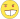For geography this is a thin wrapper around the geometry implementation. It determines a planar spatial reference system that best fits the bounding box of the geography object (trying UTM, Lambert Azimuthal Equal Area (LAEA) North/South pole, and finally Mercator ). The buffer is computed in the planar space, and then transformed back to WGS84. This may not produce the desired behavior if the input object is much larger than a UTM zone or crosses the datelineBuffer output is always a valid polygonal geometry. Buffer can handle invalid inputs, so buffering by distance 0 is sometimes used as a way of repairing invalid polygons. ST_MakeValid can also be used for this purpose.Buffering is sometimes used to perform a within-distance search. For this use case it is more efficient to use ST_DWithin.This function ignores the Z dimension. It always gives a 2D result even when used on a 3D geometry.

Enhanced: 2.5.0 - ST_Buffer geometry support was enhanced to allow for side buffering specification `side=both|left|right`.

Availability: 1.5 - ST_Buffer was enhanced to support different endcaps and join types. These are useful for example to convert road linestrings into polygon roads with flat or square edges instead of rounded edges. Thin wrapper for geography was added.

Performed by the GEOS module.This method implements the OGC Simple Features Implementation Specification for SQL 1.1.

s2.1.1.3This method implements the SQL/MM specification.

SQL-MM IEC 13249-3: 5.1.30

## Examples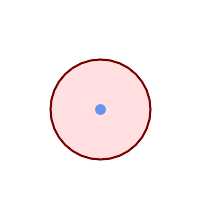quad_segs=8 (default) ```SELECT ST_Buffer( ST_GeomFromText('POINT(100 90)'), 50, 'quad_segs=8'); ```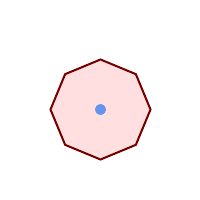quad_segs=2 (lame) ```SELECT ST_Buffer( ST_GeomFromText('POINT(100 90)'), 50, 'quad_segs=2'); ```endcap=round join=round (default) ```SELECT ST_Buffer( ST_GeomFromText( 'LINESTRING(50 50,150 150,150 50)' ), 10, 'endcap=round join=round'); ```endcap=square ```SELECT ST_Buffer( ST_GeomFromText( 'LINESTRING(50 50,150 150,150 50)' ), 10, 'endcap=square join=round'); ```endcap=flat ```SELECT ST_Buffer( ST_GeomFromText( 'LINESTRING(50 50,150 150,150 50)' ), 10, 'endcap=flat join=round'); ```join=bevel ```SELECT ST_Buffer( ST_GeomFromText( 'LINESTRING(50 50,150 150,150 50)' ), 10, 'join=bevel'); ```join=mitre mitre_limit=5.0 (default mitre limit) ```SELECT ST_Buffer( ST_GeomFromText( 'LINESTRING(50 50,150 150,150 50)' ), 10, 'join=mitre mitre_limit=5.0'); ```join=mitre mitre_limit=1 ```SELECT ST_Buffer( ST_GeomFromText( 'LINESTRING(50 50,150 150,150 50)' ), 10, 'join=mitre mitre_limit=1.0'); ```side=left ```SELECT ST_Buffer( ST_GeomFromText( 'LINESTRING(50 50,150 150,150 50)' ), 10, 'side=left'); ```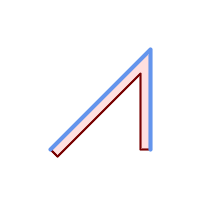side=right ```SELECT ST_Buffer( ST_GeomFromText( 'LINESTRING(50 50,150 150,150 50)' ), 10, 'side=right'); ```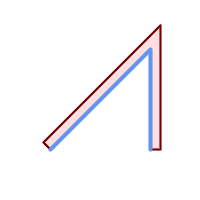side=left join=mitre ```SELECT ST_Buffer( ST_GeomFromText( 'LINESTRING(50 50,150 150,150 50)' ), 10, 'side=left join=mitre'); ```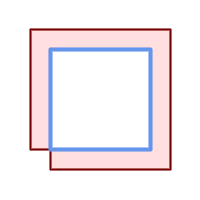right-hand-winding, polygon boundary side=left ```SELECT ST_Buffer( ST_ForceRHR( ST_Boundary( ST_GeomFromText( 'POLYGON ((50 50, 50 150, 150 150, 150 50, 50 50))'))), ), 20, 'side=left'); ```right-hand-winding, polygon boundary side=right ```SELECT ST_Buffer( ST_ForceRHR( ST_Boundary( ST_GeomFromText( 'POLYGON ((50 50, 50 150, 150 150, 150 50, 50 50))')) ), 20,'side=right') ```
```--A buffered point approximates a circle
-- A buffered point forcing approximation of (see diagram)
-- 2 points per quarter circle is poly with 8 sides (see diagram)
SELECT ST_NPoints(ST_Buffer(ST_GeomFromText('POINT(100 90)'), 50)) As promisingcircle_pcount,
ST_NPoints(ST_Buffer(ST_GeomFromText('POINT(100 90)'), 50, 2)) As lamecircle_pcount;

promisingcircle_pcount | lamecircle_pcount
------------------------+-------------------
33 |                9

--A lighter but lamer circle
-- only 2 points per quarter circle is an octagon
--Below is a 100 meter octagon
-- Note coordinates are in NAD 83 long lat which we transform
to Mass state plane meter and then buffer to get measurements in meters;
SELECT ST_AsText(ST_Buffer(
ST_Transform(
ST_SetSRID(ST_Point(-71.063526, 42.35785),4269), 26986)
,100,2)) As octagon;
----------------------
POLYGON((236057.59057465 900908.759918696,236028.301252769 900838.049240578,235
957.59057465 900808.759918696,235886.879896532 900838.049240578,235857.59057465
900908.759918696,235886.879896532 900979.470596815,235957.59057465 901008.759918
696,236028.301252769 900979.470596815,236057.59057465 900908.759918696))
```